The Distributive Property

 Problem Set 1 Problem Set 2 Problem Set 3Select a problem set using the buttons above, then use your mouse or tab key to select a question. Fill in the blank with the correct answer. Express your answers with variable terms first, in alphabetical order, followed by any constant term. Example: 2 - 3a + 2x should be expressed as -3a + 2x + 2 When you have answered all of the questions, ask Charlie how you did.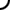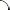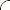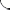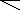Simplify each expression.   3(x + 3) - 2x 2b - 4(2b + 5) 5 - 3(6 - 2y) 4a - 2(7 - 3a) + 5 t - 4(t - 8) + 7(3t + 4) 3x + 2y - (x + 1) + 5(2y - 1)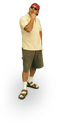Simplify each expression.   x + 4(x + 3) 5b - 7(b + 4) 14 - 2(y - 5) 9 - 3(a - 3) + 4a 8t + 5(t + 3) - 6(2t - 4) 5x + 3(2y + 1) - 2(x - 7) - 10Simplify each expression.   12 + 6(x + 2) -2b - 3(2b + 5) 4y - 8(y - 3) 6a - 2(3a - 4) + 5 -3t - (t - 3) + 7(4t + 3) -y - (3x - 3) + 8 + (4y + 9)©  2009  LarryPerezWeb Design:  Patrick Quigley